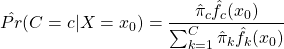## Parametric vs. Nonparametric Approach to Estimations

Parametric statistics assume that the unknown CDF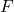belongs to a family of CDFs characterized by a parameter (vector)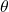. As the form ofis assumed, the target of estimation is its parameters. Thus, all uncertainty aboutis comprised of uncertainty about its parameters. Parameters are estimated by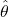, and estimates are be substituted into the assumed distribution to conduct inference for the quantities of interest. If the assumed distributionis incorrect, inference may also be inaccurate, or trends in the data may be missed.

To demonstrate the parametric approach, consider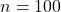independent and identically distributed random variables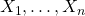generated from an exponential distribution with rate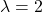. Investigators wish to estimate the 75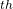percentile and erroneously assume that their data is normally distributed. Thus,is assumed to be the Normal CDF but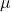and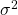are unknown. The parametersand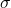are estimated in their typical way by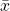and, respectively. Since the normal distribution belongs to the location-scale family, an estimate of the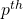percentile is provided by,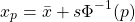where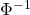is the standard normal quantile function, also known as the probit.

set.seed(12345)
library(tidyverse, quietly = T)

# Generate data from Exp(2)
x <- rexp(n = 100, rate = 2)

# True value of 75th percentile with rate = 2
true <- qexp(p = 0.75, rate = 2)
true

##  0.6931472

# Estimate mu and sigma
xbar <- mean(x)
s    <- sd(x)

# Estimate 75th percentile assuming mu = xbar and sigma = s
param_est <- xbar + s * qnorm(p = 0.75)
param_est

##  0.8792925


The true value of the 75percentile of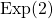is 0.69 while the parametric estimate is 0.88.

Nonparametric statistics make fewer distributions about the unknown distribution, requiring only mild assumptions such as continuity or the existence of specific moments. Instead of estimating parameters of,itself is the target of estimation.is commonly estimated by the empirical cumulative distribution function (ECDF)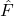,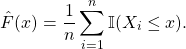Any statistic that can be expressed as a function of the CDF, known as a statistical functional and denoted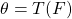, can be estimated by substitutingfor. That is, plug-in estimators can be obtained as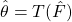.

## Motivation

It is important to have an understanding of some of the more traditional approaches to function estimation and classification before delving into the trendier topics of neural networks and decision trees. Many of these methods build on an understanding of each other and thus to truly be a MACHINE LEARNING MASTER, we’ve got to pay our dues. We will therefore start with the slightly less sexy topic of kernel density estimation.

Let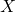be a random variable with a continuous distribution function (CDF)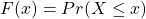and probability density function (PDF)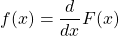Our goal is to estimate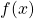from a random sample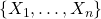. Estimation ofhas a number of applications including construction of the popular Naive Bayes classifier,